# Convection Heat Transfer Analysis with Flow Resistance for Mini-Helically Coiled Tubes at Supercritical Pressures Experimentally

Convection Heat Transfer Analysis with Flow Resistance for Mini-Helically Coiled Tubes at Supercritical Pressures Experimentally
Electromechanical Engineering Department, University of Technology, Baghdad 0096, Iraq

Corresponding Author Email:
Page:
817-824
|
DOI:
https://doi.org/10.18280/ijht.390315
23 April 2021
|
Accepted:
25 May 2021
|
Published:
30 June 2021
| Citation

OPEN ACCESS

Abstract:

This paper analyzes the effect of fluid flow characteristics on the convection heat transfer for mini-helically coiled tubes (HCT) using supercritical carbon dioxide (CO2) as a natural refrigerant. Two experimental cases have studied in this work for mini-helically coiled tubes at different diameters with different coil pitches for analyzing the convection heat transfer with flow resistance. In the first case, the inner tube diameter, coil diameter and coil pitch were 5 mm, 200 mm and 10 mm respectively, while 10 mm, 100 mm and 5 mm were for the second case. Moreover, this work has also investigated the influence of frictional pressure drop, heat flux, friction factor and mass flux on dimensionless exergy destruction. The work environments were 300-500 K as an inlet temperatures range, 200-2000 Kg / (m2. s) as a mass heat fluxes range, 50,000-500,000 as a Reynolds number (Re) range and 50-200 Kw/m2 as an inner heat fluxes range. As a result, a large effect has been observed for dimensionless exergy destruction compared with the flow friction of CO2 which induced by heat transfer irreversibility. On the other point of view, a good sensitivity of optimal Re with the tube dimeter and mass flux also noticed compared with the heat flux. At a suitable range for Re, smallest and best exergy destruction also noticed for the tube diameters. A correlation has for the optimal Reynolds number as function of main dimensionless parameters related to wall heat flux, mass flux, fluid properties and geometric dimensions is proposed. Characteristics of the fluid flow had influenced significantly by mass and heat fluxes. In the future, the collected experimental data can be employed in order to design and improve the refrigeration conditioning performance for exchangers and other systems such as heat pumps.

Keywords:

supercritical CO2, helically coiled tube, exergy destruction, friction factor and pressure drop

1. Introduction

Characteristics of supercritical CO2 (SC-CO2) as a working fluid has attracted the attention of researchers in many industrial applications due to its a natural material, Global Warming and Ozone Depletion Potentials equal to one and zero respectively, friend to the environment, non-toxic and non-flammable as well as it has low pressure and temperature than the water relatively. There are many famous systems used SC-CO2, combined systems and Organic Rankine cycle [1, 2]. Also, recent industry production has used SC-CO2 in addition to it could solve the dangerous production processes on the environment that increased nowadays . On various kinds of cotton fabrics, SC-CO2 has utilized in impregnation process by ammonium palmitate. The aim of work is to prove the effect of water-repellent, in addition to present ability of the modified and pure SC-CO2 to dissolve the ammonium palmitate [4, 5]. Jaddoa , studied experimentally the convection heat transfer for mini-tubes with fins in the presence of SC-CO2 media. The study included effect of many parameters on the convection heat transfer such as, inlet temperature, friction factor, mass flow rate, heat flux, performance evaluation criteria, enhancement efficiency and Nusselt number. In air-conditioning systems (heat pump), mini-tube as a helical coiled with SC-CO2 has employed widely in order to recover the low-grade waste heat . The reason behind that is superior the HCT performance compared with straight one in addition to its embedded structure. Thus, in heat exchangers design, its necessary to study the characteristics of heat transfer for HCT s with SC-CO2 media. Wang et al.  employed the SC-CO2 for HTC media experimentally in order to investigate the characteristics of flow. The results showed that the there is a little bit difference between frictional pressure drop for SC-CO2 and single-phase water. Where, in the beginning, it decreased with the mass flux then increased. Another contribution was made by Xu et al.  to cool the heat transfer experimentally using SC-CO2 for HCT when the wall heat flux was constant. The results analyzed according to exergy, and they reached out the optimal Re and dimensionless exergy destruction. Using simulation methods, many pervious works have been done to study the behavior of heat transfer for straight tubes with SC-CO2 [10-12]. Moreover, turbulent Prandtl has no significant effect on the heat transfer. Two shapes of tubes have proposed by Cao et al.  one was circular and the other was triangle in horizontal position to investigate the laminar heat transfer of SC-CO2 numerically through analyzing the buoyancy and fluid physical properties influence. The results showed influence of the buoyancy in heat transfer enhancement. Another study dealt with the effect of the buoyancy on the heat transfer of SC-CO2 numerically . The results predicted the low Reynolds turbulence number of k − ε model and V2F types. In addition, they recommended that the buoyancy influence could be significant on heat transfer of SC-CO2. Li and Lu  used SC-CO2/R41 to cool the heat transfer experimentally. The outcomes revealed that the relationship between HTC and the composition of R41 was opposite, where if HTC is increased, the composition of R41 will decease and vis-versa at pseudo-critical temperature (Tpc) zone. At the same region, HTC also decreases with qw increasing, but the HTC will be influenced a little by qw if bulk temperature (Tb) be far from Tpc. Mixed SC-CO2 and ethane as a working fluid has been proposed by Kravanja et al.  for cooling the heat transfer through a coiled pipe. One of the important conclusions was the mixture's higher efficiency in cooling the system compared to pure coolants. Based on previous research, it was found that the use of coiled pipe is more preferable than straight pipe, and the reason is attributed to the fact that the liquid flowing in the coiled tubes is subjected to centrifugal force, which leads to secondary flows, thus increasing additional heat transfer and lower pressure during the cross section of the pipe . Abdel-Moneim and Ali  evaluated and improved the heat transfer performance by generating the entropy and the percentage exergy destruction rate inside tubes. To obtain the optimal geometry of duct, Şahin  employed irreversibility under laminar flows with constant heat flux. The main problem that faced the designers of heat exchanger was the process of improving heat transfer was accompanied by a decrease in pressure. Therefore, it was required to find a balance between heat transfer and pressure drop through friction loss in addition to reducing reflection in the thermal system and better use of available energy. One solution has been proposed to improve the thermal system by reducing the entropy to a minimum, whose increase leads to the emergence of thermodynamic irreversibility in terms of heat transfer and fluid flow . Therefore, in this work it is suggested to use HCT in order to prevent energy destruction and generation of entropy (irreversibility) due to heat transfer. Experimental investigation of heat transfer features for vertical tube using porous media and Carbon dioxide was studded  the results appeared that the lower pseudocritical temperature (Tpc), than the inlet temperature (To) leads to reduce the coefficient of heat transfer compared with the case of the pseudocritical temperature value higher than the inlet temperatures.

In this work the heat transfer of SC-CO2 to a HCT was experimentally verified by exergy analysis. Where, in this research an analysis of the effective parameters that affect the external energy destruction was conducted in order to obtain the best design of the HCT with the best use of the available energy. In addition, the flow characteristics of CO2 inside the HCT were also verified under uniform wall heat fluxes.

2. Experimental Setup and Integrated Devices

This paper relates with studying the characteristics of SC-CO2 convection heat transfer for mini-tubes experimentally. Figure 1 show the schematic for the system, test section and actual experimental design. The experimental rig consisted of cylinder that used to provide the compressed CO2, of system included a compressed CO2 container, thermo-compressor, magnetic pump, portable chiller with cooling water bath, digital multimeter model KL-635 0C to measure the current, flowmeter type Coriolis model ALMNSO2345, manometer, data acquisition system model HS 267A to measure the voltages that crossing through test section and electrical heater, pressure gage model KLMN687T and differential pressure gage model AKLM200C, input-output electrical power measurement system and heater. Two HCT used in this work to investigated the convection heat transfer with different inner diameters (5 and 10 mm), different coil diameters (200 and 100 mm) and different coil pitches (10 and 5 mm). polytetrafluoroethylene ethylene (PTFE) layer has used for thermally and electrically insulating and locates between screws and the flanges. To heat the HCT, an electrical resistance wire has used and wrapped around it. Voltages, currents and resistance used to calculate the input electrical power for heater. Other parameters have experimentally measured such as” voltages of heater, pressure drop flow rate, pressure and temperature inlets, outlet and wall temperatures and electrical resistance”. 15 thermocouples made form copper-constantan used in this work to measure the local tube temperature and fixed by welding on the outer surface of the HCT in depth and width of 2 mm along the tube. An accurate thermal resistor used to Measure of inlet and outlet temperature after mixing the fluid before and after test section by mixers. All thermal measurement devices have been calibrated before using to obtain an accurate reading according to oil bath constant temperature with accuracy of ±0.3℃. on the other hand, many transducers have employed to measure pressure. One is the pressure gage model KLMN687T to measure the inlet pressure, and the other is differential pressure model KLM200C to measure the pressure drop. The full range and accuracy for both transduce were 15MPa, 0.04% and 800 KPa, 0.09% respectively, in order to build a sufficient imagination, mass flow rate has conducted. The accuracy of flowmeter was 0.4% and ranged from 0–100 kg/h of nominal reading. Different processes implemented before the experimental work. One of these was evacuate the test loop by vacuum pump, and the other was washed in the test loop by CO2 with a high purity of 99.9% from 6 to 8 times. To make the pressure inside the test loop steady at 5 MPa up to 10 MPa, Thermo-compressor used to feed the container and then to the test loop by CO2 which is increased gradually. The compressed CO2 remained inside it by closing the valve, and super-critical magnetic pump used to circulate CO2 at SC pressure. The test loop was tested to notice the leakage which was very small at high pressure. Moreover, silicate glass fiber and sponges used to insulate the test loop. inlet fluid temperature, mass flow rate and input power were measured at each test by connecting the measurement instruments to the personal computer by data acquisition system (HS 3986T). When the system reached to the steady state conditions, pressure drop, inlet pressure, mass flow rate and temperatures have monitored in addition to record the electrical voltage and current for heater. The term of local bulk indicates to calculate the fluid temperature at each measuring section according to the input power, mass flow rate and inlet to outlet temperatures. The heat balance uncertainty for experiments was ±6%. The system reaches to the steady state condition after 40 to 160 mins, in spite of this, changes in flow rate, temperature and inlet pressure were monitored during the initial transition. The acceptable limit of temperature variations for inlet and outlet, and wall temperature within ±0.1℃ at steady state condition, while the acceptable limit of inlet pressure and flow rater variations within ±0.2% in same condition (steady for 10 mins at least). The main reason for being the heat transfer coefficients by convection is experimentally uncertain is due to errors in the heat transfer surface temperature calculation, errors in the measurement of temperatures in addition to the experimental errors in the temperature balance and the axial thermal conductivity. The experimental uncertainty has been estimating by the root-mean square for convection heat transfer coefficient and inlet pressures which were equal to 11.3% and ±0.09% for the HCT respectively.

## 1a.png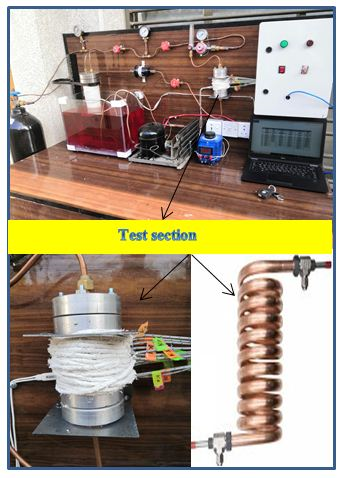(a)

## 1b.png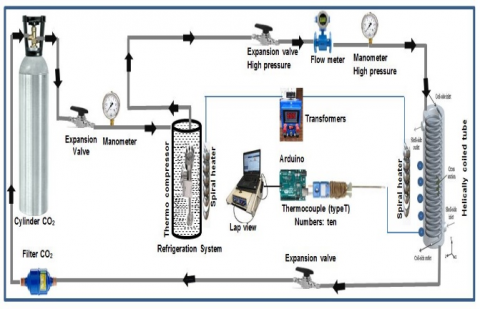(b)

## 1c.png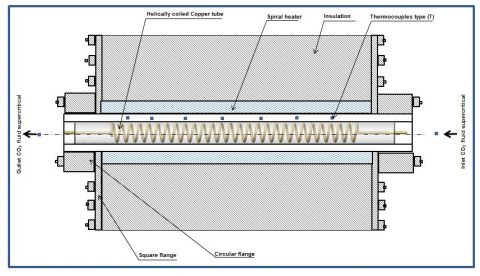(c)

Figure 1. (a) Actual experimental work of supercritical carbon dioxide; (b) schematic experimental work of supercritical carbon dioxide; (c) schematic test section

Nusselt and Reynolds numbers for HCT heat transfer in two dimensionless can be defined by:

$N u=\frac{k d}{\gamma}, R e=\frac{\rho u d}{\mu}$         (1)

where, u is referring to the average velocity and $\gamma$ refers to the heat transfer average coefficients. The equation below uses to calculate the heat transfer as:

$k=\frac{q}{\text { mean temperature }}$           (2)

mean temperature $=\frac{\left(T_{\text {in }}-T_{w i}\right)-\left(T_{\text {out }}-T_{w i}\right)}{\ln \left(\frac{T_{\text {in }}-T_{w i}}{T_{\text {out }}-T_{w i}}\right)}$                 (3)

where, $T_{w i}$ means the inner wall temperature, while $T_{\text {out,in }}$ means the outside and inside temperatures. The equation below utilized to calculate the CO2 bulk temperature of CO2 as:

$T_{b}=\frac{T_{i n}-T_{o u t}}{2}$           (4)

Eqns. (5), (6) and (7) are used to calculate the dimensionless number destruction, dimensionless exergy destruction caused by irreversibility heat transfer and dimensionless exergy destruction caused by frictional irreversibility as follows:

$N_{e}=\ln \frac{1-6 S T \varphi \omega}{1-\omega-4 S T \omega \varphi}+\frac{\ln (1-6 \omega \varphi) E S}{12 S T}$               (5)

$N_{h}=\ln \frac{1-6 S T \varphi \omega}{1-\omega-4 S T \omega \varphi}$             (6)

$N_{f}=\ln \frac{1-E S(1-6 \omega \varphi S T)}{12 S T}$                (7)

where, ST refers to the Station number which equal to $S T=\frac{N_{u}}{R_{e} P_{r}}$, ES refers to the Eskert number which equal to $S T=\frac{u^{2}}{C_{p}\left(\frac{q}{h}\right)^{2}}, \varphi$  refers to the dimensionless length passage of the coil (l/d) and $\omega$ refers to the dimensionless inlet temperature ratio. Eq. (8) clears how to calculate the total pressure drop as:

pressure drop $=$ friction pressure drop $+$ gravitational pressure drop + acceleration pressure drop      (8)

$\left(\Delta p_{f}\right)=\left(\Delta p_{m}\right)-\left(\Delta p_{g}\right)+\left(\rho_{o} g h-\Delta p_{a}\right)$         (9)

Eq. (10) used to calculate the Darcy friction factor is as:

$f_{c}=\frac{6 d \Delta p_{f} \rho_{o}}{G^{3} L}$            (10)

3. Results and Discussion

Due to this paper focuses on studying the exergy destruction and heat transfer performance at pseudo-critical zone, Figure 2 presents the CO2 thermo-physical properties at 8 MPa of pressure. CO2 thermo-physical properties consisting of Cp which is the specific heat, λ which is the thermal conductivity, ρ which is the fluid density and μ which is the dynamic viscosity. CO2 thermo-physical properties dramatically changing near the Tpc. It can be seen at 8 MPa that the CO2 critical temperature was equal to 307.8 K approximately.

## 2.png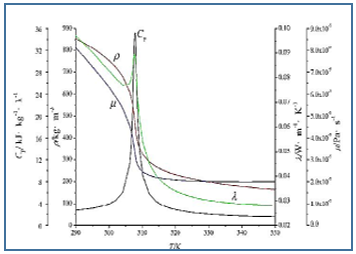Figure 2. Thermo-physical properties of CO2 under the pressure of 8 MPa

## 3.pngFigure 3. Nh and Nf performance with Re at d=10mm G=250 Kg / m2.s and q=150 kW/m2

Also, it important to study the thermodynamic analysis and interfacing between Nf and Nh due to the irreversibilities resultant by friction and heat transfer which considering one of the important tasks for HCT. At G=250 Kg / m2.s and q=150 kW/m2 when the tube diameter is 10 mm, Figure 3 presents the comparison between Nf and Nh. the main observation in this figure was the values of Nh always bigger than Nf when the region in turbulent flow which was different at water and air phenomenon. The loss by irreversibility that resultant by friction factor be higher than heat transfer influence with increasing of Re. In Eq. (6) which present the ration of irreversibility distribution to illustrate the Nh and Nf results, it can see in flow field that the exergy destruction was mainly resulting by heat transfer irreversibility. In addition, it can neglect the effect of dimensionless exergy destruction caused by flow friction for heat transfer when the HCT be cooled. The decreasing in CO2 viscosity led to high decreasing in dimensionless exergy destruction caused by flow friction. Figure 3 also indicates at turbulent flow, curve of the Nh arrived to the lowest value for heat transfer of HCT, and optimal Re can be obtained at lowest dimensionless exergy destruction. The biggest. value of Ne was bigger than lowest Ne about 45 times. This concludes that the optimal HCTs design according to the principle of dimensionless exergy destruction minimization is very important for the exergy utilization of thermal systems.

Figure 4 shows the heat fluxes variations (100-200 Kw/m) with the dimensionless exergy destruction which is considering as a function of bulk temperature when the values of P and G equal to 8 MPa and 250 Kg/m.s respectively. Moreover, at pseudo-critical point, the curve of Ne reached to the lowest value at various heat fluxes. The reason behind that was the enhancement in heat transfer at the account of the highest specific heat value. Another notice according to the irreversibility, entropy generation and exergy destruction being in the same trend of variation. The main indication can be seen in pseudo-critical region was the big difference in gas-like and liquid-like regions with different heat fluxes. Therefore, heat transfer enhancement led the value of dimensionless exergy destruction near to 0.01. In addition, increase in the heat fluxes leads to the increase of the dimensionless exergy destruction due to weak the heat transfer as a result of the big difference between bulk and wall temperatures.

## 4.pngFigure 4. Dimensionless exergy destruction and temperature bulk Performance for various heat flux

The relationship between different mass fluxes (250, 500, 1000, 1500 and 2000 Kg/m2s) and dimensionless exergy destruction has presented in Figure 5 when the q=100 kW/m2 and P=8 MPa. This figure proved that the lowest dimensionless exergy destruction value was near Tpc. With increasing of mass flux, enhancement the heat transfer performance due to the turbulent diffusion has increased and lead to decrease the exergy destruction.

## 5.png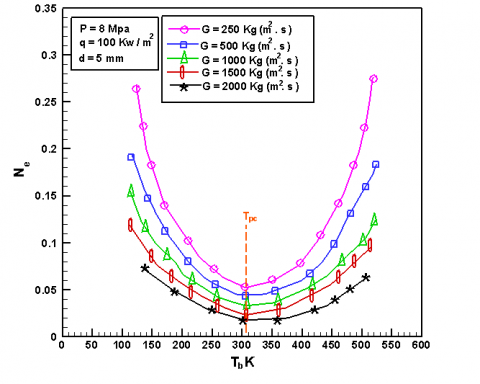Figure 5. Dimensionless exergy destruction and temperature bulk Performance for various mass fluxes

Figure 6 presents different pressure with different the dimensionless exergy destruction variation. The dimensionless exergy destruction increased with increasing the pressure in addition of the lower value of dimensionless exergy destruction noticed at Tpc. Also, bulk temperature increased at 8 MPa to 10 MPa of pressure range due to thermo-physical properties variation. Moreover, the point above Tpc lead to increase the pressure and decease the dimensionless exergy destruction compared with the point below Tpc that be opposite behavior.

In order to obtain the optimal HCT design, this work also focused on the optimal factor of Re. At G=250 kg/ m2.s, Figures 7 and 8 show the experimental results of Ne and Re when the s tube diameters are 5 and 10 mm respectively. both of Figures proved that the optimal Re and lowest value of dimensionless exergy destruction which increased with heat fluxes increasing. Or, lowest Ne and optimal Re depended on heat fluxes.

At q=100 kW/m2 and d=5 mm, both of Figures 9 and 10 Present mass flux effect on optimal Re. mass flux variation have been noticed with the lowest Ne and optimal Re. according to Figures 7-10, sensitivity of Re with mass flux variation was bigger than neat flux. Where, optimal Re increased with increasing of mass flux, while lowest Ne was decreased with increasing of mass flux due to turbulent intensity.

## 6.pngFigure 6. Dimensionless exergy destruction variation at different pressures

## 7.pngFigure 7. Dimensionless exergy destruction variation for different heat flux at d=5 mm

## 8.png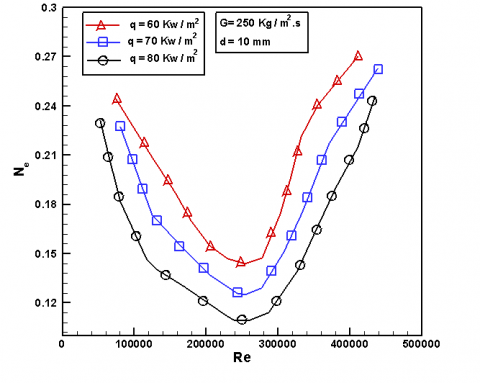Figure 8. Dimensionless exergy destruction variation for different heat flux at d=10 mm

## 9.png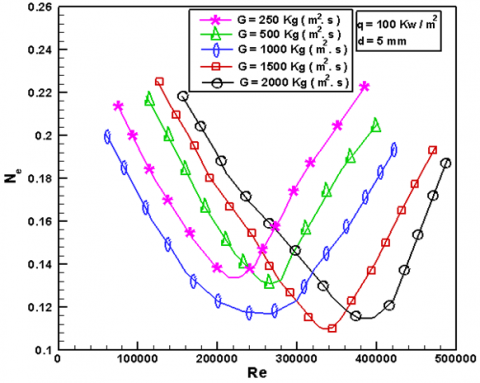Figure 9. Dimensionless exergy destruction variation for different mass heat flux at d=5 mm

## 10.png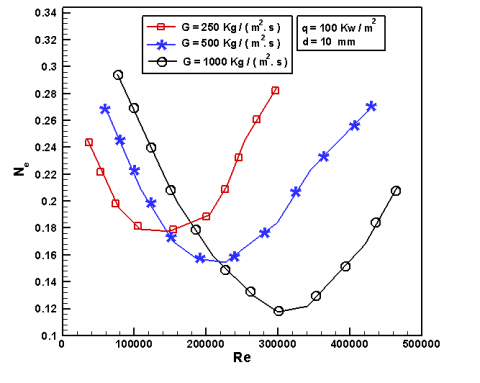Figure 10. Dimensionless exergy destruction variation for different mass heat flux at d=10 mm

Figure 11 shows the different Ne with Re at to diameters (5 and 10 mm) when G=250 kg/ m2.s and q=100 kW/m2. Smaller values of Ne have noticed in proper Re range and best exergy utilization for both diameters. dimensionless exergy destructions have increased with dimeter increasing when Re<150000, but opposite behavior has been noticed when Re>350000. Moreover, smallest dimensionless exergy destruction has noticed when the diameter was 5 mm compared with the diameter of 10 mm.

## 11.png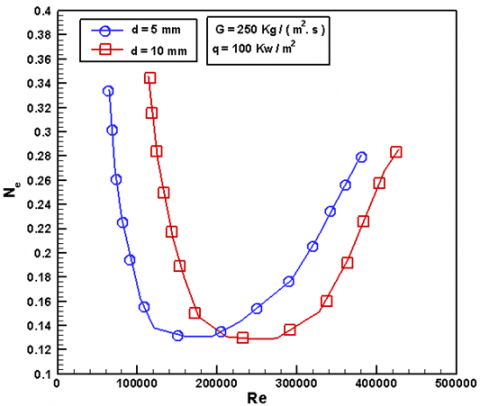Figure 11. Re with dimensionless exergy destruction variation

According to the previous investigations, increasing of optimal Re depends on the increasing in heat flux, tube diameter and mass flux. Also, a correlation must be inferred to establish the relationship between both quantities.

$10^{-8} \mathrm{Re}=1.986 \times 10^{-9} \alpha^{0.633} \beta^{-2.564} \gamma^{3.345}$               (11)

Correlation is an important part in the prediction of the optimal Reynolds number based on the appropriate operating condition with the parameters determined for the shape of HCT. It should take into consideration that the correlation deals with turbulent convective heat transfer in HCT when the wall heat flux is constant.

Figure 11 shows the relationship between varied heat fluxes (70 to 90 kW/m2) and pressure at 8.0 MPa. While, Figure 12 presents sing-phase water for friction pressure drop with mass fluxes. The main indication for Figure 12 was the frictional pressure drop increases with increasing of mass fluxes due to larger velocity gradient and results a shear stress for wall. When the value of heat flux equal to zero, the frictional pressure drop keep monotonically increasing with rising mass flux. The frictional pressure drop decreases firstly and then increases at q=80 kW/m2. There is a small changing in frictional pressure drop between 70 and 80 kW/m2.

## 12.png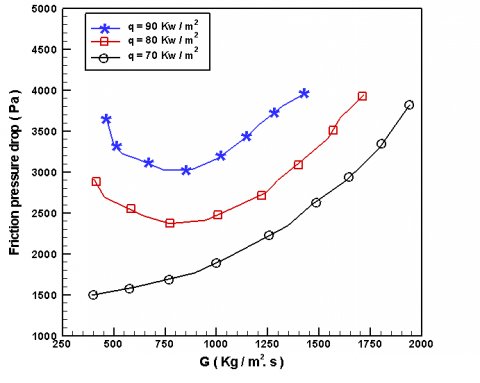Figure 12. Mass fluxes with friction pressure drop variation

Figure 13 shows the mass flux variation from 100 to 1500 kg/m2.s when the operating pressure was fixed at 8.0 MPa, while Figure 14 shows friction pressure drop variation with heat fluxes. According to Figure 14, when the heat fluxes increase, the frictional pressure drop decreases due to decreasing the viscosity with increasing of temperature which in turn decreasing the shear stress of wall in addition to physical properties variation dramatically (especially the density) near Tpc which leads to decreasing the frictional pressure drop and increasing the heat fluxes. The reasons behind decreasing the density and increasing the frictional pressure drop increase are; firstly, the thermal acceleration leads to decrease the density near wall region and producing large shear stress and frictional pressure drop. Finally, difference in density throughout the test tube leads to the flow resistance by causing a secondary flow which is perpendicular to the main flow direction.

## 13.png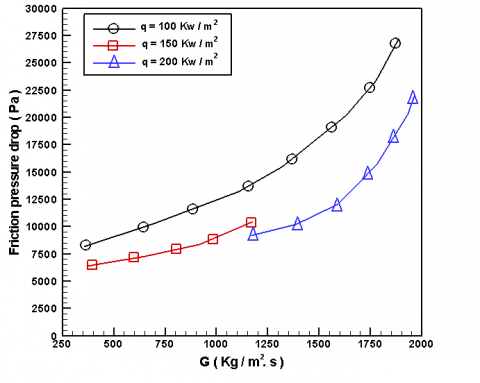Figure 13. Mass fluxes with friction pressure drop variation for single- phase water

## 14.pngFigure 14. Heat fluxes with friction pressure drop variation for CO2

Figures 15 and 16 describes the CO2 friction factor behavior with heat fluxes for HCT. Its increased gradually with heat fluxes. friction factor has reached to the maximum value. At Tpc, the fluid temperature was near the wall, CO2 transferred into the supercritical liquid. The reason that led the friction factor for increasing was reduction the density that worked on the accelerate the flow and increasing the shear stress. While, the reason led the friction factor into decreasing after that was due to the fluid passing through Tpc.

Friction factor behavior with Re number can be seen in Figure 17 in addition to describing the Ito and white correlations. The main notice according to Figure 17 was decreasing of friction diagram with increasing of Re which is the same behavior in straight pipe case and the value was higher than which calculated by the two equations. Indeed, the empirical formula was depended on the flow condition. single-phase water used to obtain Ito and white correlations as a test fluid in HCT.

## 15.png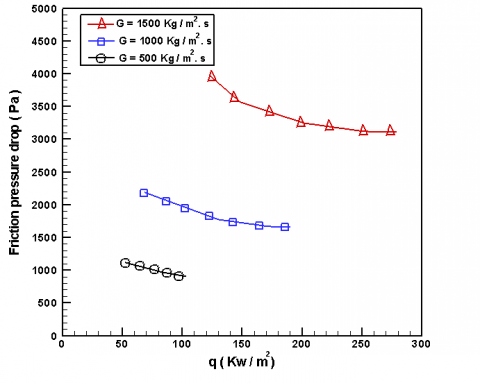Figure 15. Heat fluxes with friction pressure drop variation for single- phase water

## 16.png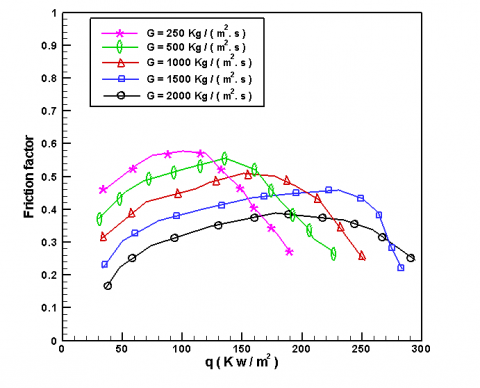Figure 16. Friction factor variation with heat fluxes

## 17.png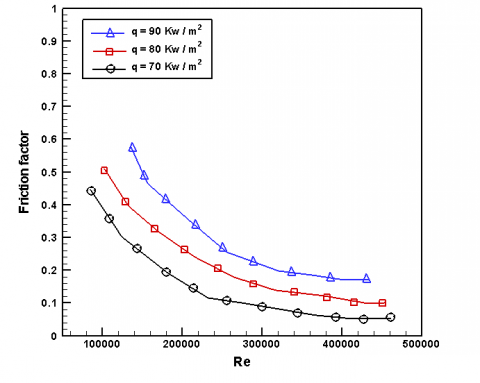Figure 17. Friction factor variation with Re

4. Conclusion

Analysis of exergy for HCT experimentally through study the heat transfer for supercritical CO2 has been conducted in this work. Many parameters described the system behavior which are: mass and heat fluxes, tube diameters, frictional factor and frictional pressure drop. Investigation in this paper was done based on analyzing the dimensionless exergy destruction and the optimal Reynolds number influences. Based on pervious discussions. heat transfer irreversibility was led to generate the dimensionless exergy destruction which was higher than the flow friction for SC-HCT. Optimal Re and minimum dimensionless exergy destruction have been reached. The tube diameter of 5 mm gave a smaller dimensionless exergy destruction than the tube diameter of 10 mm when 150000<Re<350000. Tube diameter and mass fluxes have a great effect on optimal Re more than heat fluxes. optimal Re has predicted by proposed correlation in optimal operation conditions to for heat transfer in SC-HCT in order to design optimal HCT. The frictional pressure drop increased with mass fluxes firstly the decreased for supercritical CO2 with small different than single-phase water. Finally, when the mass fluxed was fixed, the frictional pressure drop decreased with the heat flux.

Nomenclature
 Nu Nusselt number $N u=\frac{k d}{\gamma}$ Re Reynolds number $R e=\frac{\rho u d}{\mu}$ u Average velocity m/s k coefficients of heat transfer (Wm-2k-1) $T_{w i}$ Inner wall temperature (K) $T_{\text {out }, \text { in }}$ Outside and inside temperature (K) $T_{b}$ bulk temperature of CO2 (K) $N_{e}$ dimensionless number destruction $N_{h}$ dimensionless exergy destruction caused by heat transfer irreversibility $N_{f}$ dimensionless exergy destruction caused by frictional irreversibility ST Station number $S T=\frac{N_{u}}{R_{e} P_{r}}$ ES Eskert number $S T=\frac{u^{2}}{C_{p}\left(\frac{q}{h}\right)}$ $\Delta P$ Pressure drop (Mpa) d Diameter of tube (mm) u Velocity of fluid (m.s-1) l Pitch of coil (mm) Cp specific heat capacity J/(K. kg) G Mass flux (Kg/m2.s) q Heat flux Kw/m2 p Pressure (Mpa) T Temperature (K) Greek symbols $\gamma$ Average coefficients of heat transfer $\varphi$ dimensionless ratio of Inlet and outlet temperature $\omega$ Inlet temperature dimensionless ratio $\alpha$ Length of Dimensionless passage of the coil (l/d) $\beta$ Dimensionless duty parameter $\frac{q}{G C_{P} T_{i n}}$ $\gamma$ difference ratio of dimensionless temperature inlet $f$ friction factor Subscripts in Inlet out Outlet w wall i inside o outside
References

 Tian, H., Chang, L., Shu, G., Shi, L. (2017). Multi-objective optimization of the carbon dioxide transcritical power cycle with various configurations for engine waste heat recovery. Energy Conversion and Management, 148: 477-488. https://doi.org/10.1016/j.enconman.2017.05.038

 Xu, X.X., Liu, C., Fu, X., Gao, H., Li, Y. (2015). Energy and exergy analyses of a modified combined cooling, heating, and power system using supercritical CO2. Energy, 86: 414-422. https://doi.org/10.1016/j.energy.2015.04.043

 Tian, H., Wu, M., Shu, G., Liu, Y., Wang, X. (2017). Experimental and theoretical study of flammability limits of hydrocarbon–CO2 mixture. International Journal of Hydrogen Energy, 42(49): 29597-29605. https://doi.org/10.1016/j.ijhydene.2017.10.053

 Bilalov, T.R., Zakharov, A.A., Jaddoa, A.A., Gumerov, F.M., Le Neindre, B. (2017). Treatment of different types of cotton fabrics by ammonium palmitate in a supercritical CO2 environment. The Journal of Supercritical Fluids, 130: 47-55. https://doi.org/10.1016/j.supflu.2017.07.036

 Gumerov, F.M., Le Neindre, B., Bilalov, T.R., Sagdeev, A.A. (2016). Regeneration of spent catalyst and impregnation of catalyst by supercritical fluid. International Journal of Analytical Mass Spectrometry and Chromatography, 4(4): 51-65. https://doi.org/10.4236/ijamsc.2016.44006

 Jaddoa, A.A. (2021). Convection heat transfer performance for the SCF-CO2 media in mini-tube with fins experimentally. Journal of Engineering Science and Technology.

 Naphon, P., Wongwises, S. (2006). A review of flow and heat transfer characteristics in curved tubes. Renewable and Sustainable Energy Reviews, 10(5): 463-490. https://doi.org/10.1016/j.rser.2004.09.014

 Wang, S.X., Zhang, W., Xu, J.L. (2013). Experimental investigation on flow characteristics of supercritical CO2 in a helically coiled tube. In Applied Mechanics and Materials, 368(370): 631-635. https://doi.org/10.4028/www.scientific.net/AMM.368-370.631

 Xu, X., Zhang, Y., Liu, C., Zhang, S., Dang, C. (2018). Experimental investigation of heat transfer of supercritical CO2 cooled in helically coiled tubes based on exergy analysis. International Journal of Refrigeration, 89: 177-185. https://doi.org/10.1016/j.ijrefrig.2018.03.011

 Launder, B.E., Sharma, B.I. (1974). Application of the energy-dissipation model of turbulence to the calculation of flow near a spinning disc. Letters in Heat and Mass Transfer, 1(2): 131-137. https://doi.org/10.1016/0735-1933(74)90024-4

 Dang, C., Hihara, E. (2004). In-tube cooling heat transfer of supercritical carbon dioxide. Part 2. Comparison of numerical calculation with different turbulence models. International Journal of Refrigeration, 27(7): 748-760.

 Dang, C., Hihara, E. (2004). In-tube cooling heat transfer of supercritical carbon dioxide. Part 1. Experimental measurement. International Journal of Refrigeration, 27(7): 736-747. https://doi.org/10.1016/j.ijrefrig.2004.04.018

 Cao, X.L., Rao, Z.H., Liao, S.M. (2011). Laminar convective heat transfer of supercritical CO2 in horizontal miniature circular and triangular tubes. Applied Thermal Engineering, 31(14-15): 2374-2384. https://doi.org/10.1016/j.applthermaleng.2011.03.038

 He, S., Kim, W.S., Jackson, J.D. (2008). A computational study of convective heat transfer to carbon dioxide at a pressure just above the critical value. Applied Thermal Engineering, 28(13): 1662-1675. https://doi.org/10.1016/j.applthermaleng.2007.11.001

 Li, M.X., Lu. J.T. (2015). Heat transfer characteristics of supercritical CO2/R41 flowing in mini-channel. CIESC Journal, 66(3): 924-931.

 Kravanja, G., Zajc, G., Knez, Ž., Škerget, M., Marčič, S., Knez, M.H. (2018). Heat transfer performance of CO2, ethane and their azeotropic mixture under supercritical conditions. Energy, 152: 190-201. https://doi.org/10.1016/j.energy.2018.03.146

 Cioncolini, A., Santini, L. (2006). On the laminar to turbulent flow transition in diabatic helically coiled pipe flow. Experimental Thermal and Fluid Science, 30(7): 653-661. https://doi.org/10.1016/j.expthermflusci.2006.01.004

 Abdel-Moneim, S.A., Ali, R.K. (2007). Performance evaluation of heat transfer enhancement for internal flow based on exergy analysis. International Journal of Exergy, 4(4): 401-420. https://doi.org/10.1504/IJEX.2007.015081

 Şahin, A.Z. (1998). Irreversibilities in various duct geometries with constant wall heat flux and laminar flow. Energy, 23(6): 465-473. https://doi.org/10.1016/S0360-5442(98)00010-3

 Bejan, A. (1996). Entropy generation minimization: The new thermodynamics of finite-size devices and finite-time processes. Journal of Applied Physics, 79(3): 1191-1218. https://doi.org/10.1063/1.362674

 Kkihlefa, B.J., Gaddoa, A.A., Reja, A.H. (2021). Experimental investigation of heat transfer features for vertical tube using porous media and Carbon dioxide, Journal of Mechanical Engineering Research and Developments, 44(5): 188-195.